# Recurrence Networks

A recurrence network is a representation of a recurrence matrix as a graph (a network). The nodes in the network represent time points, and if a value at any point in time will recur at some later point in time, an edge is drawn between the time points.

## Recurrence Networks

Recurrence networks are graphs created from a recurrence matrix. This means the nodes of the graph represent time points and the connections between nodes represent a recurrence relation betwen the values observed at those time points. That is, often the matrix represents recurrences in a reconstructed state space, the values are coordinates and therefore we would say the edges of a recurrence network represent a temporal relation between recurring states. The ultimate reference for learning everything about recurrence networks is:

Zou, Y., Donner, R. V., Marwan, N., Donges, J. F., & Kurths, J. (2018). Complex network approaches to nonlinear time series analysis. Physics Reports

Package casnet has some functions to create recurrence networks, they are similar to the functions used for CRQA: * rn() is very similar to rp(), it will create a matrix based on embedding parameters. One difference is the option to create a weighted matrix. This is a matrix in which non-recurring values are set to 0, but the recurring values are not replaced by a 1, the distance value is retained and acts as an edge-weight * rn_plot() will produce the same as rp_plot()

We can turn the recurrence matrix into an adjecency matrix, an igraph object. This means we can use all the igraph functions to calculate network measures.

library(igraph)
#library(qgraph)
#library(survival)
# Reload the data we used earlier
series <- rio::import("https://github.com/complexity-methods/CSA-assignments/raw/master/assignment_data/BasicTSA_arma/series.xlsx")

# Lets use a shorter dataset to speed things up
series <- series[1:500,]

We’ll analyse the three time series as a recurrence network: * Compare the results by comparing the different measures - Remember: TS_1 was white noise, TS_2 was a sine with added noise, TS_3 was the logistic map in the chaotic regime. * Note that some of the RQA measures can be exactly calculated from the measures of the network representation. - Try to understand why the Recurrence is represented as the degree centrality of the network (igraph::centr_degree())

### TS 1

#----------------------
#----------------------
plot(ts(series$TS_1))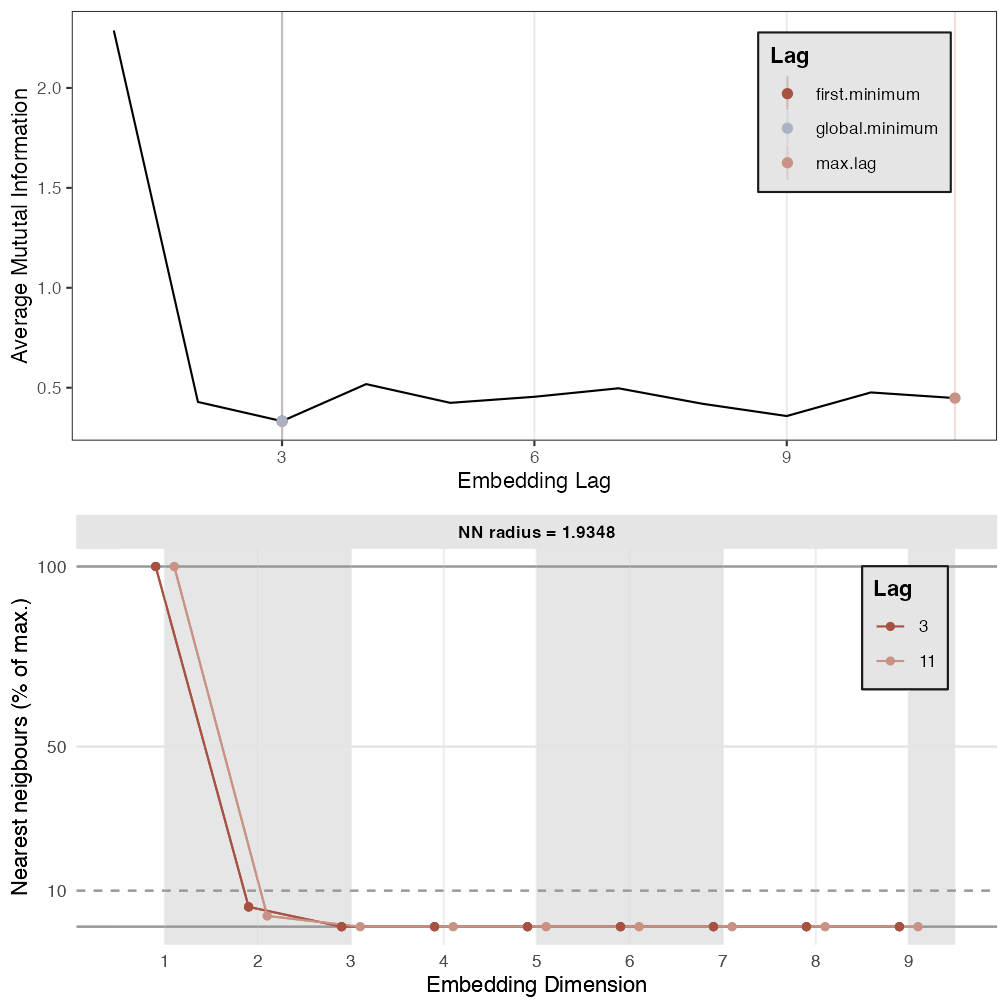arcs <- 6 # Because these are generated signals, look for a drop in FNN below 1%. p1 <- est_parameters(y = series$TS_1, nnThres = 1)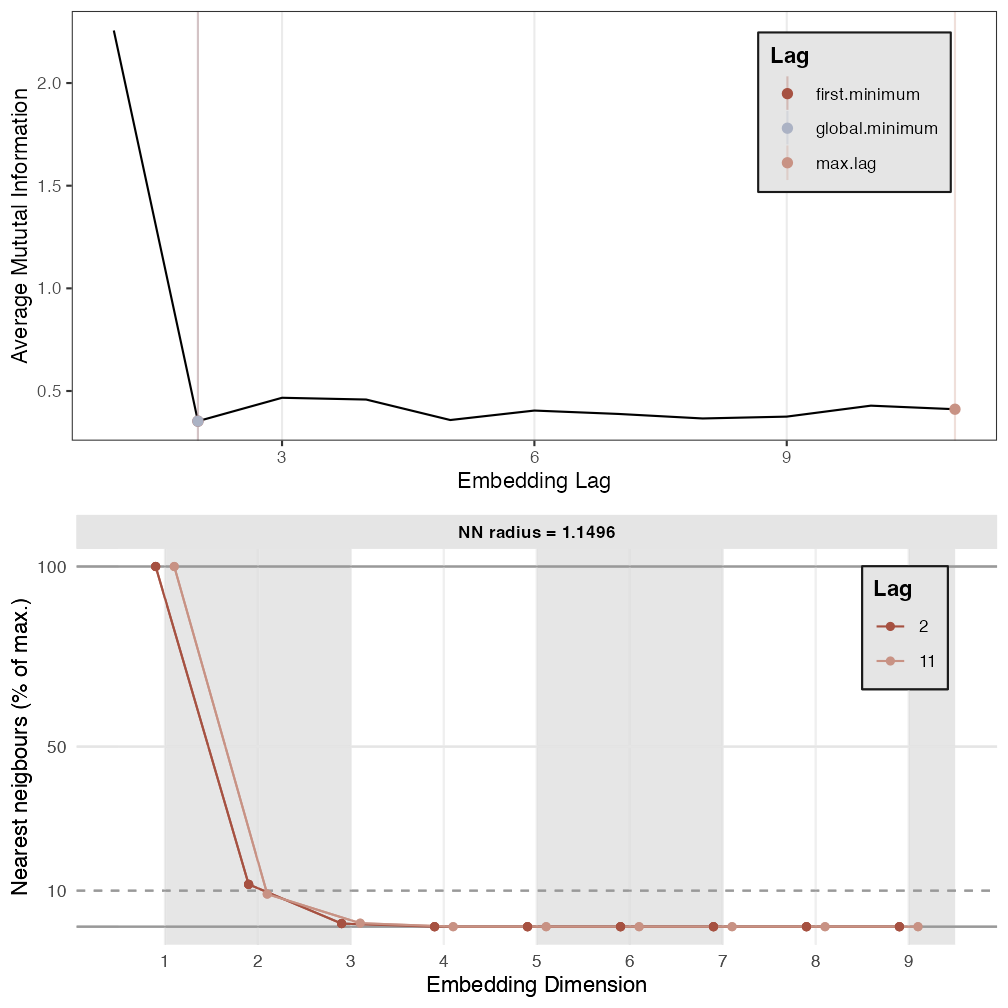RN1 <- casnet::rn(y1 = series$TS_1, emDim = p1$optimDim, emLag = p1$optimLag, emRad = NA, targetValue = 0.05) > > Auto-recurrence: Setting diagonal to (1 + max. distance) for analyses > > Searching for a radius that will yield 0.05 for RR casnet::rn_plot(RN1)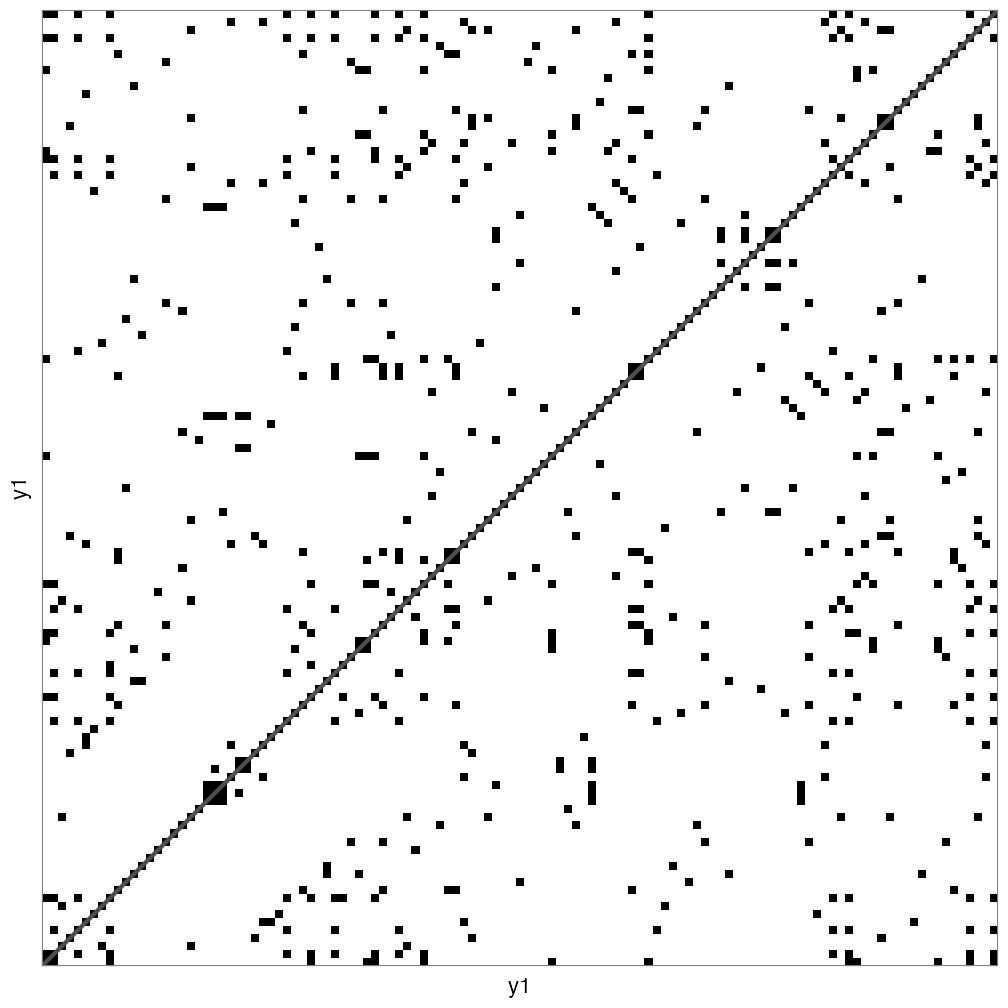# Get RQA measures rqa1 <- casnet::rp_measures(RN1, silent = FALSE) > > ~~~o~~o~~casnet~~o~~o~~~ > > Global Measures > Global Max.rec.points N.rec.points Recurrence.Rate Singular.points > 1 Recurrence Matrix 244530 12228 0.05000613 11140 > Divergence Repetitiveness Anisotropy > 1 0.1428571 1.58364 1 > > > Line-based Measures > Line.based N.lines N.points.on.lines Measure Rate Mean Max > 1 Diagonal 420 1088 Determinism 0.08897612 2.590476 7 > 2 Vertical 774 1723 V Laminarity 0.14090612 2.226098 6 > 3 Horizontal 774 1723 H Laminarity 0.14090612 2.226098 6 > Entropy.of.lengths Relative.entropy CoV.of.lengths > 1 1.0355136 0.16695004 0.3890404 > 2 0.5764252 0.09293379 0.2592375 > 3 0.5764252 0.09293379 0.2592375 > > ~~~o~~o~~casnet~~o~~o~~~ # Create RN graph g1 <- igraph::graph_from_adjacency_matrix(RN1, mode="undirected", diag = FALSE) igraph::V(g1)$size <- igraph::degree(g1)
g1r <- casnet::make_spiral_graph(g1,arcs = arcs, epochColours = getColours(arcs), markTimeBy = TRUE)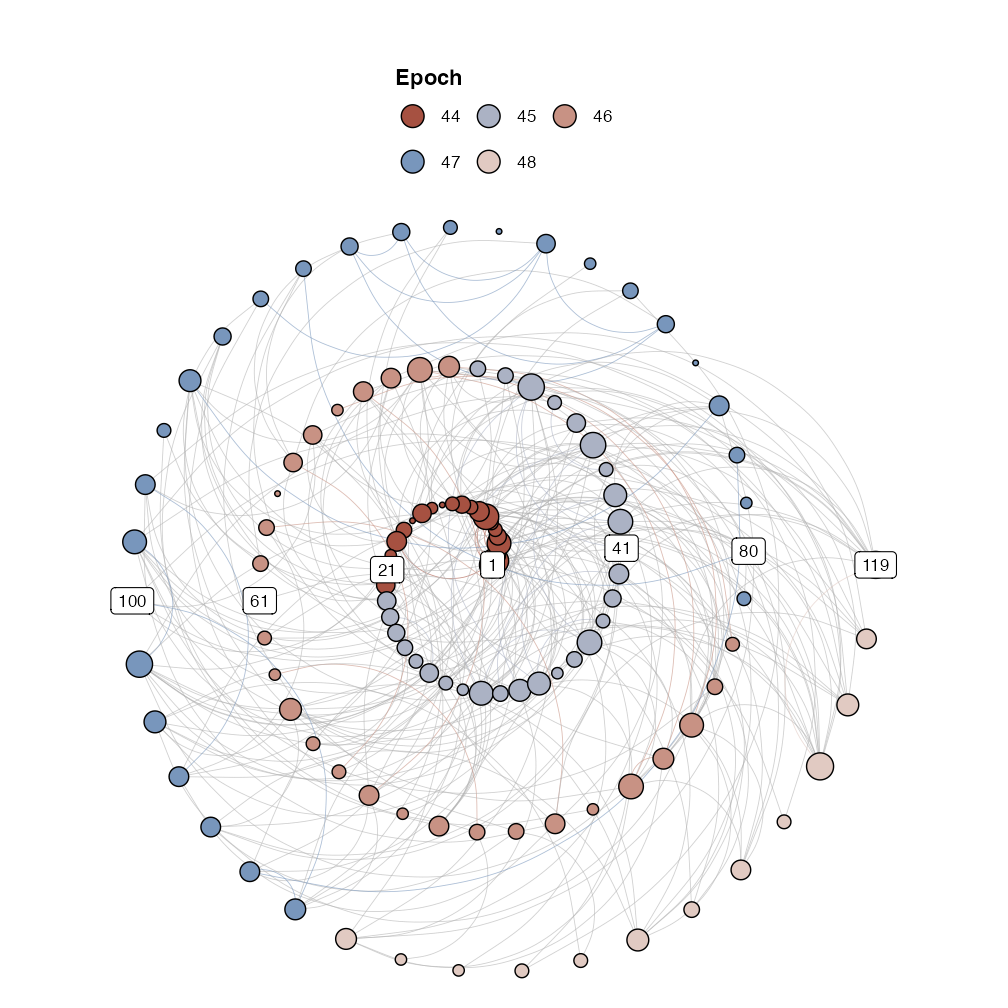# Get RN measures
rn1 <- rn_measures(g1, silent = FALSE)
>
> ~~~o~~o~~casnet~~o~~o~~~
>
> Global Network Measures
>
>   EdgeDensity MeanStrengthDensity GlobalClustering NetworkTransitivity
> 1  0.05000613                  NA        0.5104217           0.5161686
>   AveragePathLength GlobalEfficiency
> 1          51.95484         3.212035
>
> ~~~o~~o~~casnet~~o~~o~~~

# Should be the same
rqa1$RR==rn1$graph_measures$EdgeDensity >  TRUE ### TS 2 #---------------------- # Adjacency matrix TS_2 #---------------------- plot(ts(series$TS_2))# Because these are generated signals, look for a drop in FNN below 1%.
p2 <- est_parameters(y = series$TS_2, nnThres = 1)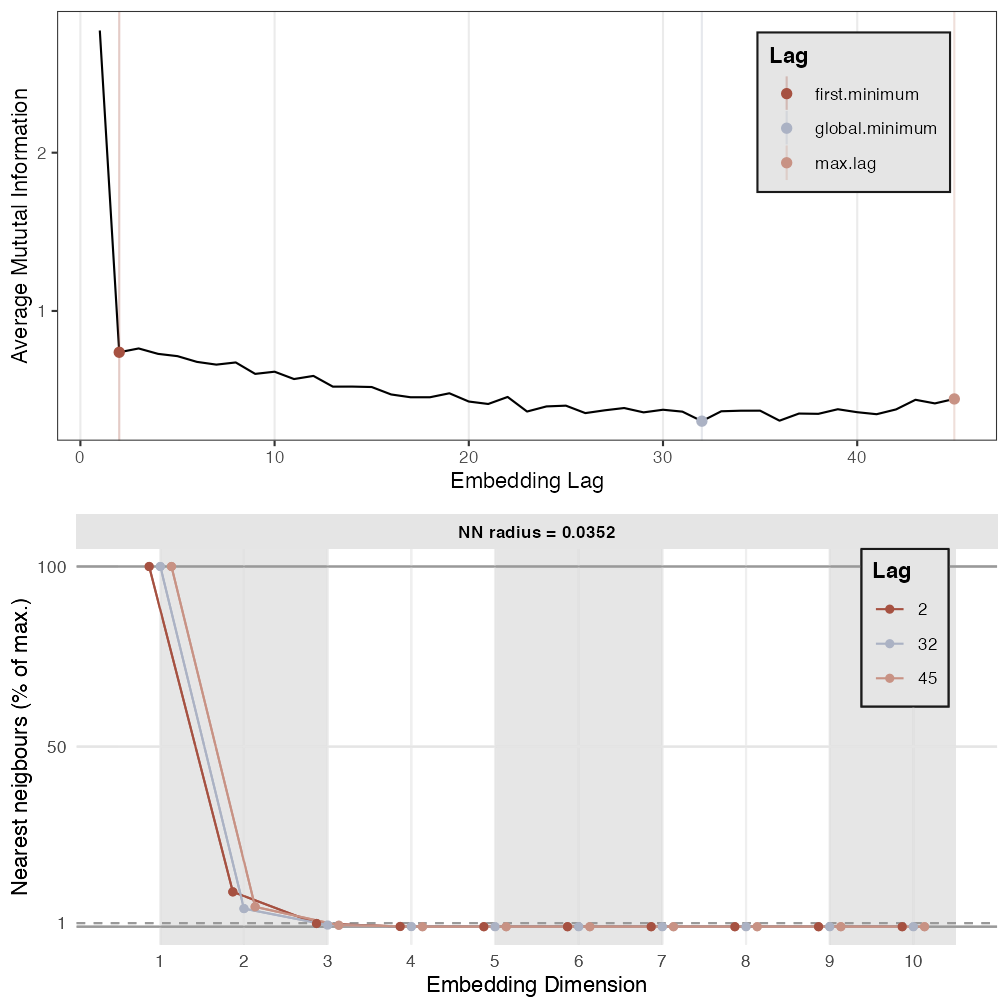RN2 <- rn(y1 = series$TS_2, emDim = p2$optimDim, emLag = p2$optimLag, emRad = NA,  targetValue = 0.05)
>
> Auto-recurrence: Setting diagonal to (1 + max. distance) for analyses
>
> Searching for a radius that will yield 0.05 for RR
rn_plot(RN2)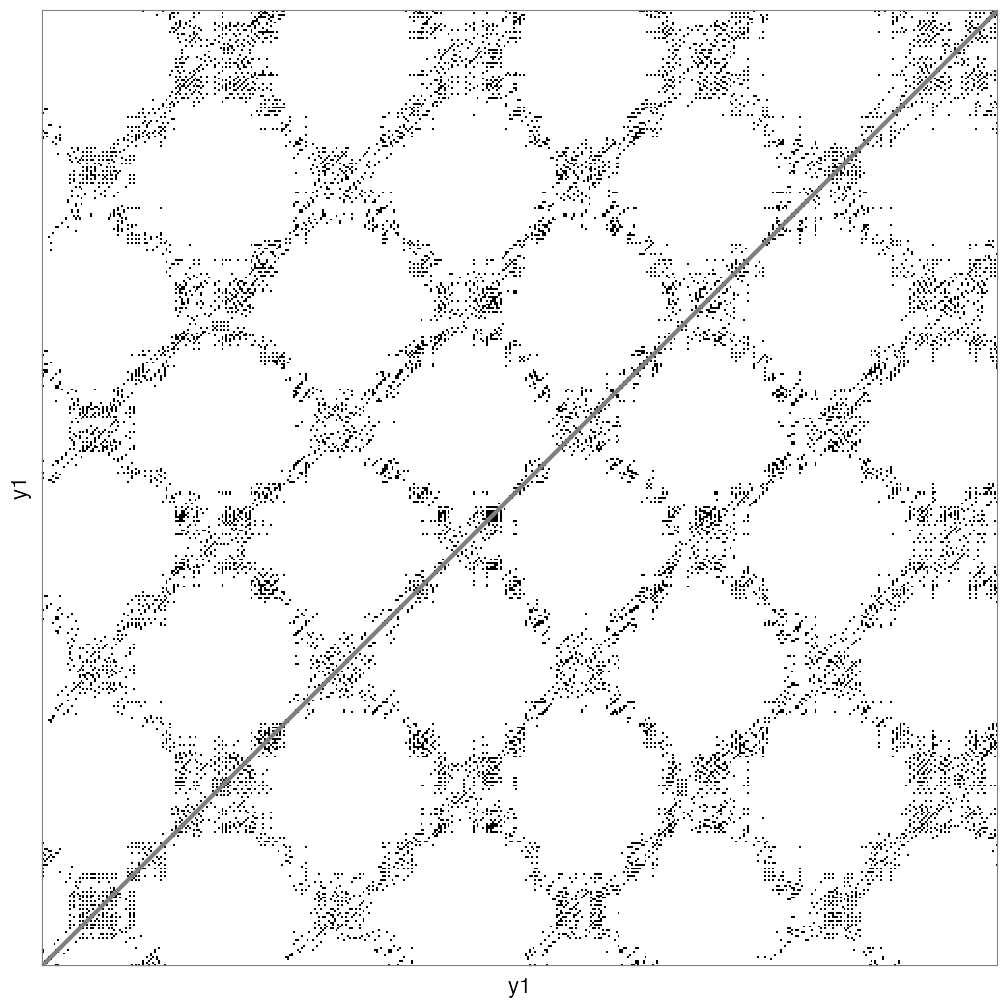# Get RQA measures
rqa2 <- rp_measures(RN2, silent = FALSE)
>
> ~~~o~~o~~casnet~~o~~o~~~
>
>  Global Measures
>              Global Max.rec.points N.rec.points Recurrence.Rate Singular.points
> 1 Recurrence Matrix         244530        12228      0.05000613            9874
>   Divergence Repetitiveness Anisotropy
> 1  0.1111111        1.52294          1
>
>
>  Line-based Measures
>   Line.based N.lines N.points.on.lines      Measure      Rate     Mean Max
> 1   Diagonal     804              2354  Determinism 0.1925090 2.927861   9
> 2   Vertical    1552              3585 V Laminarity 0.2931796 2.309923   8
> 3 Horizontal    1552              3585 H Laminarity 0.2931796 2.309923   8
>   Entropy.of.lengths Relative.entropy CoV.of.lengths
> 1          1.3217260        0.2130945      0.4538389
> 2          0.7052368        0.1137014      0.3101225
> 3          0.7052368        0.1137014      0.3101225
>
> ~~~o~~o~~casnet~~o~~o~~~

# Create RN graph
g2 <- igraph::graph_from_adjacency_matrix(RN2, mode="undirected", diag = FALSE)
V(g2)$size <- degree(g2) g2r <- make_spiral_graph(g2,arcs = arcs ,epochColours = getColours(arcs), markTimeBy = TRUE)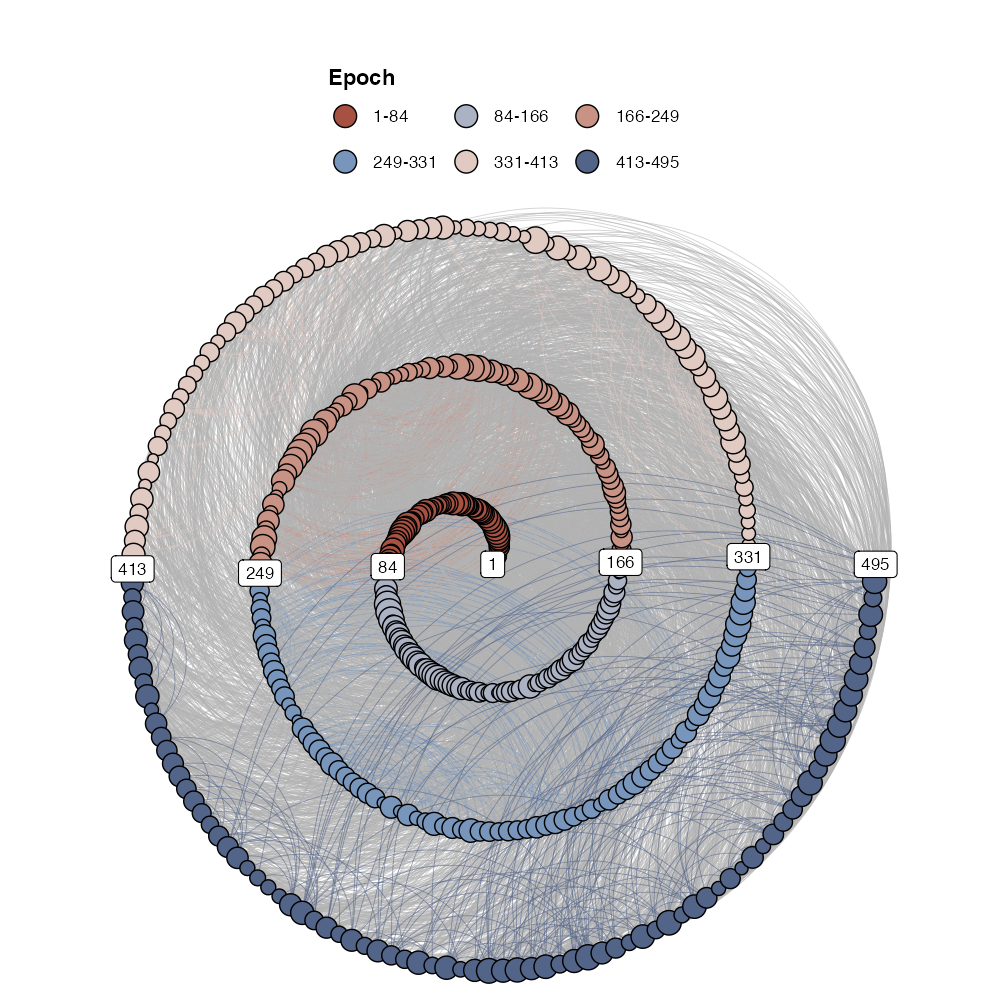# Get RN measures rn2 <- rn_measures(g2, silent = FALSE) > > ~~~o~~o~~casnet~~o~~o~~~ > > Global Network Measures > > EdgeDensity MeanStrengthDensity GlobalClustering NetworkTransitivity > 1 0.05000613 NA 0.5781826 0.5369779 > AveragePathLength GlobalEfficiency > 1 7.020586 3.510234 > > ~~~o~~o~~casnet~~o~~o~~~ # Should be the same rqa2$RR==rn2$graph_measures$EdgeDensity
>  TRUE

### TS 3

#----------------------
#----------------------
plot(ts(series$TS_3))# Because these are generated signals, look for a drop in FNN below 1%. p3 <- est_parameters(y = series$TS_3, nnThres = 1)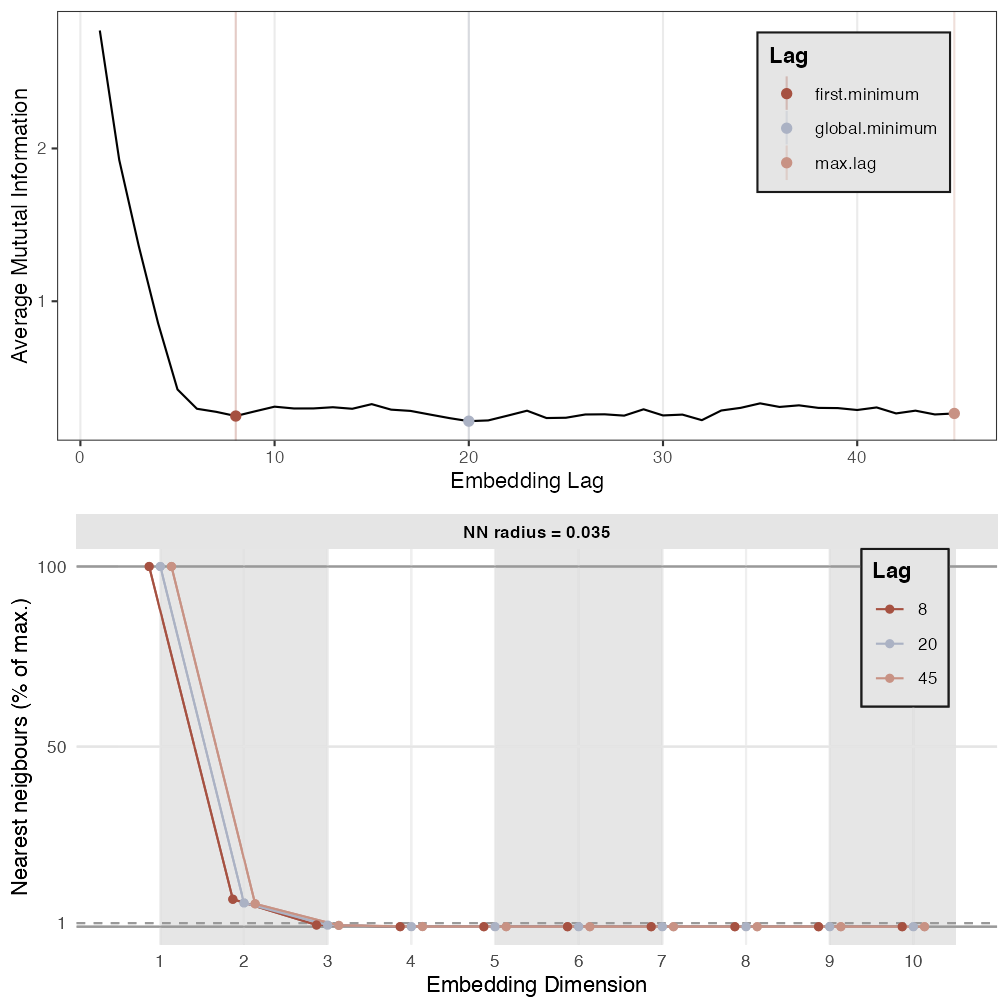RN3 <- rn(y1 = series$TS_3, emDim = p3$optimDim, emLag = p3$optimLag, emRad = NA, targetValue = 0.05) > > Auto-recurrence: Setting diagonal to (1 + max. distance) for analyses > > Searching for a radius that will yield 0.05 for RR rn_plot(RN3)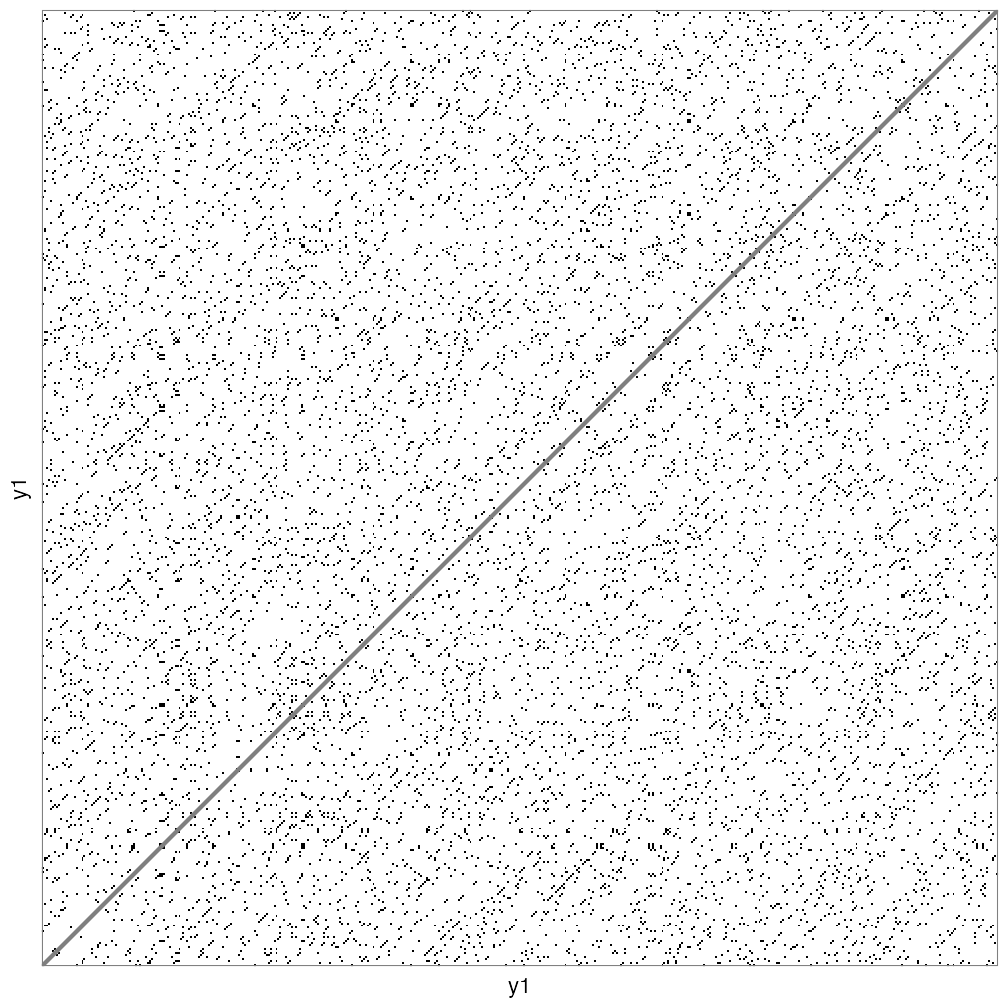# Get RQA measures rqa3 <- rp_measures(RN3, silent = FALSE) > > ~~~o~~o~~casnet~~o~~o~~~ > > Global Measures > Global Max.rec.points N.rec.points Recurrence.Rate Singular.points > 1 Recurrence Matrix 232806 11642 0.0500073 7662 > Divergence Repetitiveness Anisotropy > 1 0.1666667 0.170603 1 > > > Line-based Measures > Line.based N.lines N.points.on.lines Measure Rate Mean Max > 1 Diagonal 1776 3980 Determinism 0.34186566 2.240991 6 > 2 Vertical 324 679 V Laminarity 0.05832331 2.095679 3 > 3 Horizontal 324 679 H Laminarity 0.05832331 2.095679 3 > Entropy.of.lengths Relative.entropy CoV.of.lengths > 1 0.6092768 0.09862128 0.2508340 > 2 0.3154837 0.05106613 0.1405776 > 3 0.3154837 0.05106613 0.1405776 > > ~~~o~~o~~casnet~~o~~o~~~ # Create RN graph g3 <- igraph::graph_from_adjacency_matrix(RN3, mode="undirected", diag = FALSE) V(g3)$size <- degree(g3)
g3r <- make_spiral_graph(g3,arcs = arcs ,epochColours = getColours(arcs), markTimeBy = TRUE)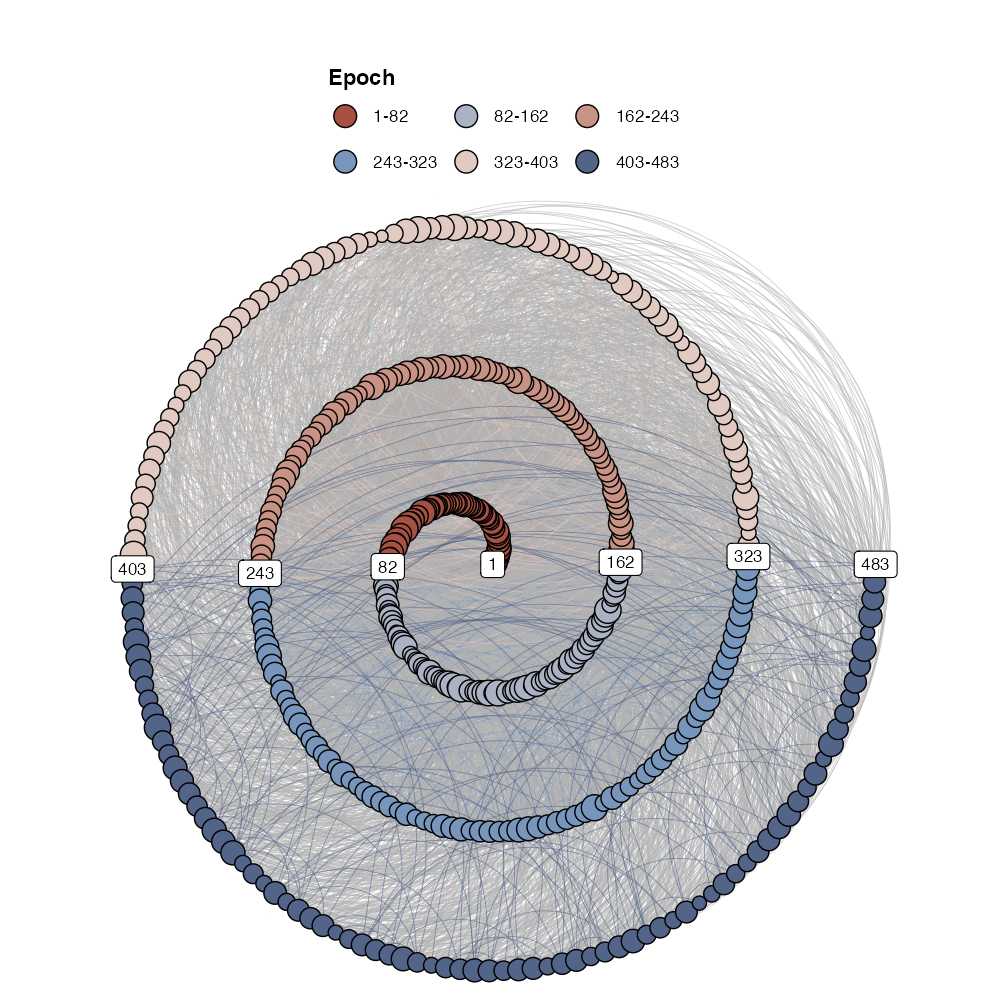# Get RN measures
rn3 <- rn_measures(g3, silent = FALSE)
>
> ~~~o~~o~~casnet~~o~~o~~~
>
> Global Network Measures
>
>   EdgeDensity MeanStrengthDensity GlobalClustering NetworkTransitivity
> 1   0.0500073                  NA        0.6030504            0.597613
>   AveragePathLength GlobalEfficiency
> 1          4.010532         3.260776
>
> ~~~o~~o~~casnet~~o~~o~~~

# Should be the same
rqa3$RR==rn3$graph_measures$EdgeDensity >  TRUE ## Multiplex Recurrence Networks Consider the three time series to be part of a multi-layer recurrence network. Common properties of the multiplex network are inter-layer mutual information and edge overlap can be calculated using function casnet::mrn(). One problem, the networks have to be all of the same size (same number of nodes, a multivariate time series), but here we have reconstructed the phase space using different embedding parameters… let’s choose one set of parameters for all time series. emDim <- mean(c(p1$optimDim,p2$optimDim,p3$optimDim))
emLag <- median(c(p1$optimLag,p2$optimLag,p3$optimLag)) RNs <- plyr::llply(1:3, function(r) rn(y1 = series[,r], emDim = emDim, emLag = emLag, emRad = NA, targetValue = 0.05)) > > Auto-recurrence: Setting diagonal to (1 + max. distance) for analyses > > Searching for a radius that will yield 0.05 for RR > > Auto-recurrence: Setting diagonal to (1 + max. distance) for analyses > > Searching for a radius that will yield 0.05 for RR > > Auto-recurrence: Setting diagonal to (1 + max. distance) for analyses > > Searching for a radius that will yield 0.05 for RR layers <- plyr::llply(RNs, function(r) igraph::graph_from_adjacency_matrix(r, mode="undirected", diag = FALSE)) names(layers) <- c("g1","g2","g3") mrn(layers = layers) > > ~~~o~~o~~casnet~~o~~o~~~ > > Welcome to the multiplex... in layer similarity mode! > > > ~~~o~~o~~casnet~~o~~o~~~ >$interlayerMI
> $interlayerMI$window: 1 | start: 1 | stop: 495
>    g1        g2        g3
> g1 NA 0.2609506 0.3133915
> g2 NA        NA 1.4864990
> g3 NA        NA        NA
>
>
> $edgeOverlap >$edgeOverlap$window: 1 | start: 1 | stop: 495 > g1 g2 g3 > g1 NA 0.02568631 0.06124579 > g2 NA NA 0.02625123 > g3 NA NA NA > > >$meanValues
> $meanValues$window: 1 | start: 1 | stop: 495
>     mi_mean     mi_sd    eo_mean      eo_sd eo_sum eo_all    eo_joint
> 1 0.6869471 0.6929286 0.03772777 0.02036916  18572     34 0.001830713
>
>
> $Nsize >  495 A variety of plots can be created using casnet::mrn_plot()  # Simple mrn_plot(layers = layers, showEdgeColourLegend =TRUE) > > ~~~o~~o~~casnet~~o~~o~~~ > > Welcome to the multiplex... in layer similarity mode! > > > ~~~o~~o~~casnet~~o~~o~~~ >$MRN
> $MRN$window: 1 | start: 1 | stop: 495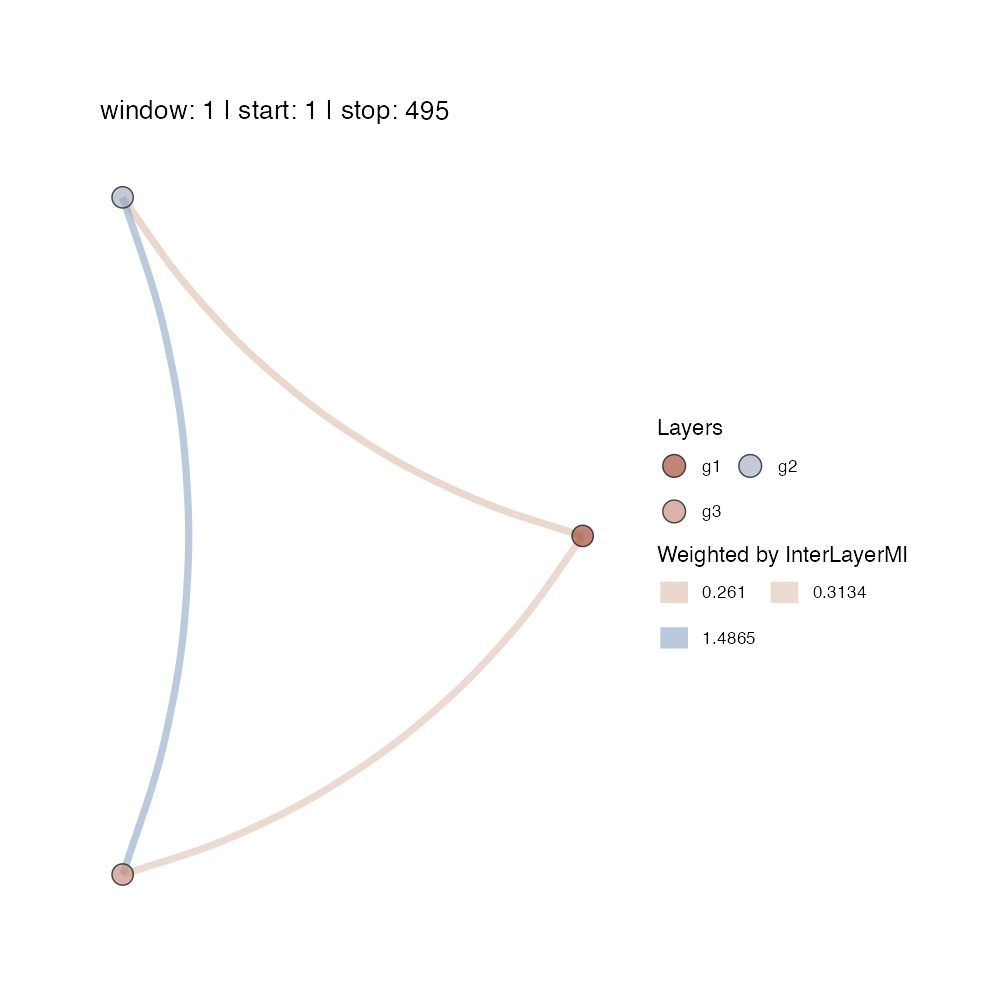>
>
> $interlayerMI >$interlayerMI$window: 1 | start: 1 | stop: 495 > g1 g2 g3 > g1 NA 0.2609506 0.3133915 > g2 NA NA 1.4864990 > g3 NA NA NA > > >$edgeOverlap
> $edgeOverlap$window: 1 | start: 1 | stop: 495
>    g1         g2         g3
> g1 NA 0.02568631 0.06124579
> g2 NA         NA 0.02625123
> g3 NA         NA         NA
>
>
> $meanValues > NULL mrn_plot(layers = layers, MRNweightedBy = "EdgeOverlap", showEdgeColourLegend =TRUE) > > ~~~o~~o~~casnet~~o~~o~~~ > > Welcome to the multiplex... in layer similarity mode! > > > ~~~o~~o~~casnet~~o~~o~~~ >$MRN
> $MRN$window: 1 | start: 1 | stop: 495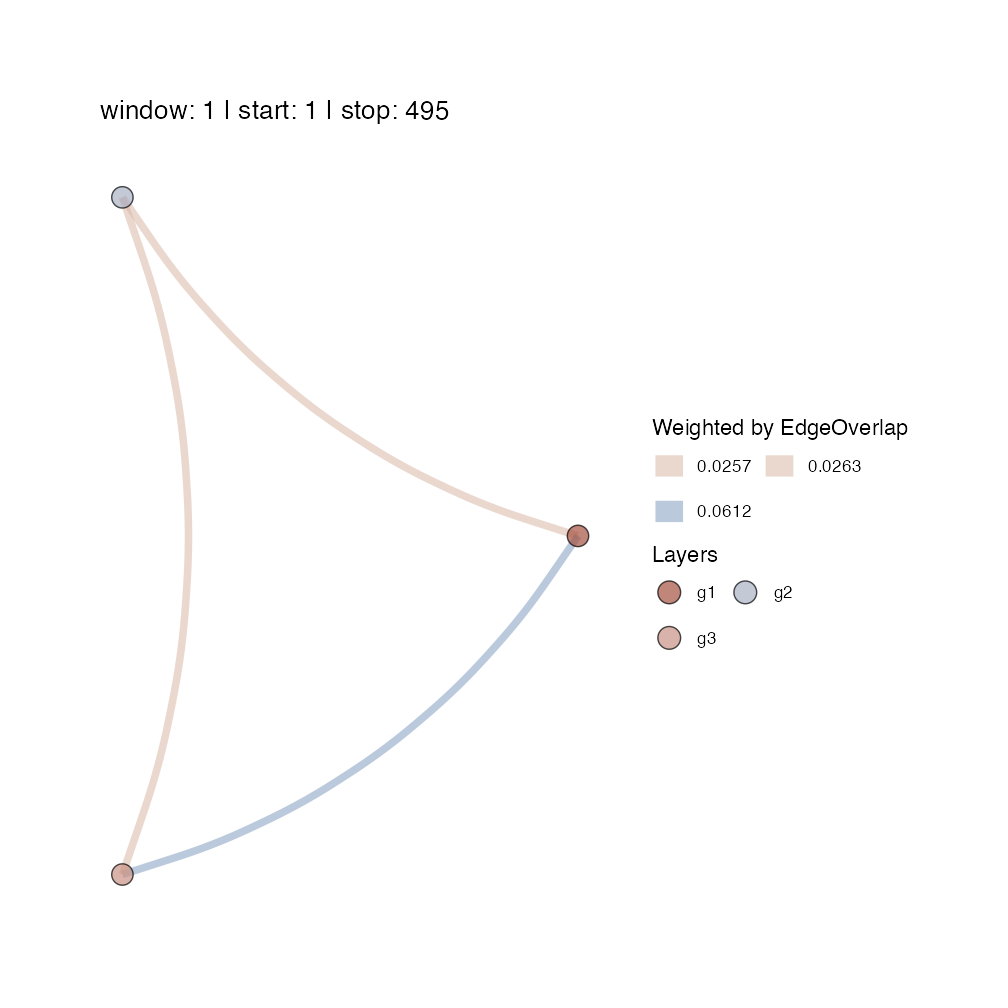>
>
> $interlayerMI >$interlayerMI$window: 1 | start: 1 | stop: 495 > g1 g2 g3 > g1 NA 0.2609506 0.3133915 > g2 NA NA 1.4864990 > g3 NA NA NA > > >$edgeOverlap
> $edgeOverlap$window: 1 | start: 1 | stop: 495
>    g1         g2         g3
> g1 NA 0.02568631 0.06124579
> g2 NA         NA 0.02625123
> g3 NA         NA         NA
>
>
> $meanValues > NULL # Include picture of Layers mrn_plot(layers = layers, RNnodes = TRUE) > > ~~~o~~o~~casnet~~o~~o~~~ > > Welcome to the multiplex... in layer similarity mode! > > > ~~~o~~o~~casnet~~o~~o~~~ >$MRN
> $MRN$window: 1 | start: 1 | stop: 495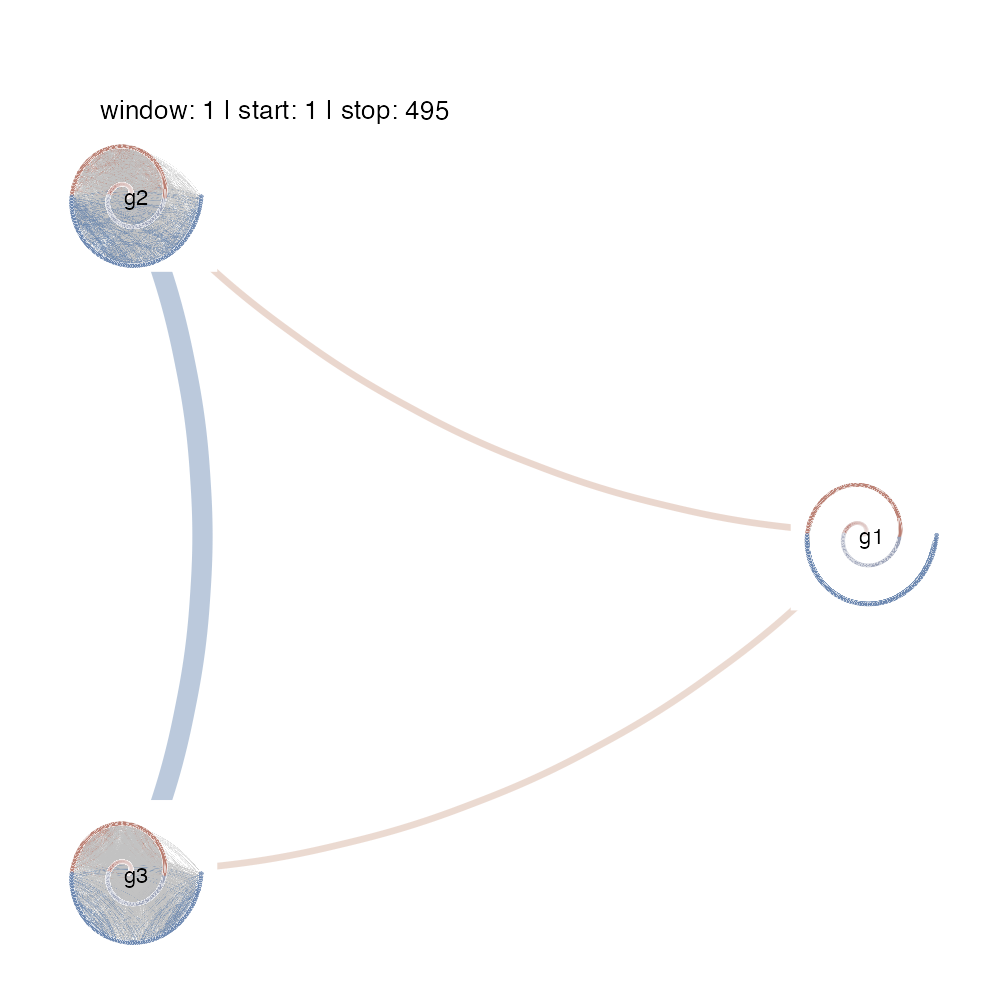>
>
> $interlayerMI >$interlayerMI$window: 1 | start: 1 | stop: 495 > g1 g2 g3 > g1 NA 0.2609506 0.3133915 > g2 NA NA 1.4864990 > g3 NA NA NA > > >$edgeOverlap
> $edgeOverlap$window: 1 | start: 1 | stop: 495
>    g1         g2         g3
> g1 NA 0.02568631 0.06124579
> g2 NA         NA 0.02625123
> g3 NA         NA         NA
>
>
> $meanValues > NULL mrn_plot(layers = layers, RNnodes = TRUE,MRNweightedBy = "EdgeOverlap", showEdgeColourLegend =TRUE) > > ~~~o~~o~~casnet~~o~~o~~~ > > Welcome to the multiplex... in layer similarity mode! > > > ~~~o~~o~~casnet~~o~~o~~~ >$MRN
> $MRN$window: 1 | start: 1 | stop: 495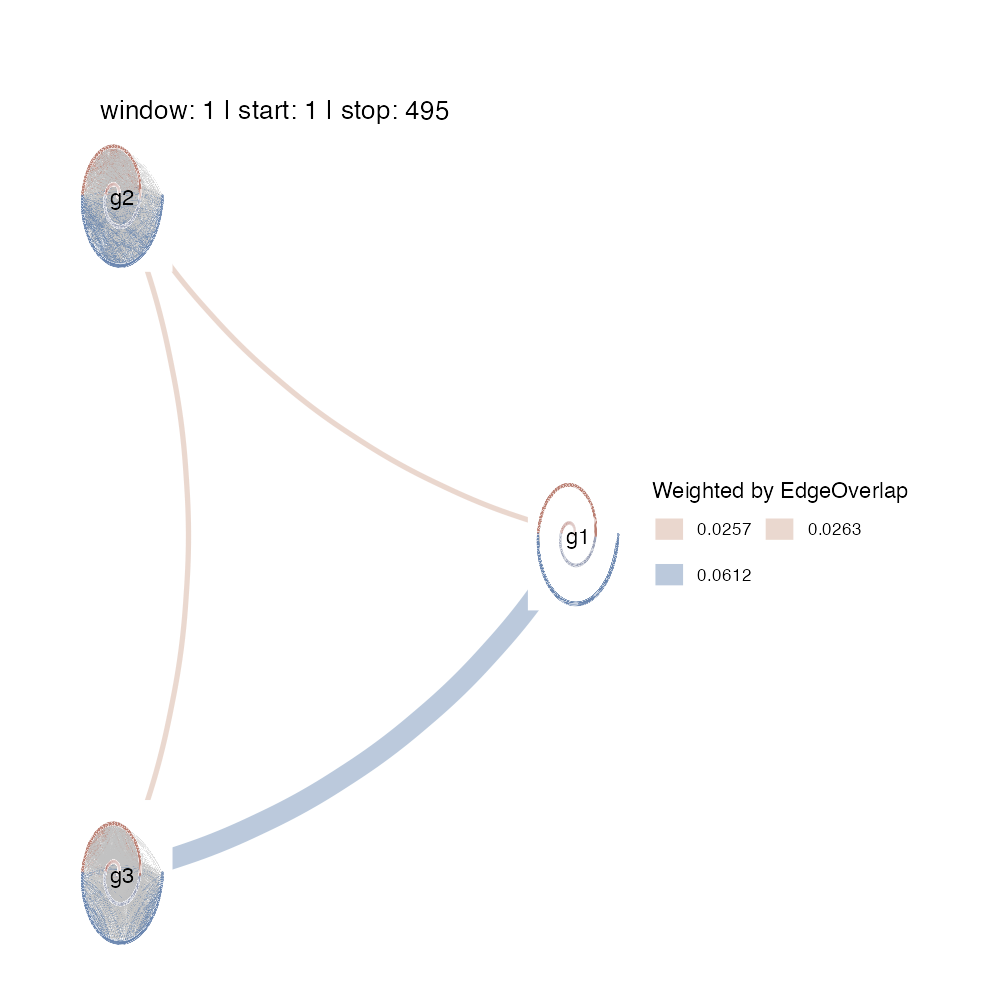>
>
> $interlayerMI >$interlayerMI$window: 1 | start: 1 | stop: 495 > g1 g2 g3 > g1 NA 0.2609506 0.3133915 > g2 NA NA 1.4864990 > g3 NA NA NA > > >$edgeOverlap
> $edgeOverlap$window: 1 | start: 1 | stop: 495
>    g1         g2         g3
> g1 NA 0.02568631 0.06124579
> g2 NA         NA 0.02625123
> g3 NA         NA         NA
>
>
> $meanValues > NULL ## Time-varying Multiplex Recurrence Networks The MRN can be calculated in a sliding window, by setting the arguments win and step.  # This will generate 26 windows MRN_win <- mrn(layers = layers, win = 250, step = 10) > > ~~~o~~o~~casnet~~o~~o~~~ > > Welcome to the multiplex... in layer similarity mode! > > > ~~~o~~o~~casnet~~o~~o~~~ # The MRN are returned as a list MRN_win$interlayerMI
> $window: 01 | start: 1 | stop: 250 > g1 g2 g3 > g1 NA 0.3377082 0.5216094 > g2 NA NA 1.6939426 > g3 NA NA NA > >$window: 02 | start: 11 | stop: 260
>    g1        g2        g3
> g1 NA 0.2687605 0.5649311
> g2 NA        NA 1.6881942
> g3 NA        NA        NA
>
> $window: 03 | start: 21 | stop: 270 > g1 g2 g3 > g1 NA 0.3708465 0.4491544 > g2 NA NA 1.6464434 > g3 NA NA NA > >$window: 04 | start: 31 | stop: 280
>    g1       g2        g3
> g1 NA 0.368284 0.4455847
> g2 NA       NA 1.7394935
> g3 NA       NA        NA
>
> $window: 05 | start: 41 | stop: 290 > g1 g2 g3 > g1 NA 0.3445478 0.5649311 > g2 NA NA 1.8526006 > g3 NA NA NA > >$window: 06 | start: 51 | stop: 300
>    g1        g2        g3
> g1 NA 0.2969798 0.5897381
> g2 NA        NA 1.8290879
> g3 NA        NA        NA
>
> $window: 07 | start: 61 | stop: 310 > g1 g2 g3 > g1 NA 0.475031 0.3404266 > g2 NA NA 1.6741508 > g3 NA NA NA > >$window: 08 | start: 71 | stop: 320
>    g1        g2        g3
> g1 NA 0.4140999 0.5532296
> g2 NA        NA 1.8609670
> g3 NA        NA        NA
>
> $window: 09 | start: 81 | stop: 330 > g1 g2 g3 > g1 NA 0.5824751 0.4637912 > g2 NA NA 1.9291099 > g3 NA NA NA > >$window: 10 | start: 91 | stop: 340
>    g1        g2        g3
> g1 NA 0.3726358 0.4256365
> g2 NA        NA 1.6247560
> g3 NA        NA        NA
>
> $window: 11 | start: 101 | stop: 350 > g1 g2 g3 > g1 NA 0.3427359 0.5163049 > g2 NA NA 2.0026265 > g3 NA NA NA > >$window: 12 | start: 111 | stop: 360
>    g1        g2        g3
> g1 NA 0.5663371 0.5236299
> g2 NA        NA 1.7002084
> g3 NA        NA        NA
>
> $window: 13 | start: 121 | stop: 370 > g1 g2 g3 > g1 NA 0.4577888 0.5714332 > g2 NA NA 1.7548832 > g3 NA NA NA > >$window: 14 | start: 131 | stop: 380
>    g1       g2        g3
> g1 NA 0.424447 0.5894762
> g2 NA       NA 1.7602788
> g3 NA       NA        NA
>
> $window: 15 | start: 141 | stop: 390 > g1 g2 g3 > g1 NA 0.673275 0.5958021 > g2 NA NA 1.9566326 > g3 NA NA NA > >$window: 16 | start: 151 | stop: 400
>    g1        g2        g3
> g1 NA 0.5742539 0.6372795
> g2 NA        NA 1.9088293
> g3 NA        NA        NA
>
> $window: 17 | start: 161 | stop: 410 > g1 g2 g3 > g1 NA 0.6137142 0.559716 > g2 NA NA 1.882073 > g3 NA NA NA > >$window: 18 | start: 171 | stop: 420
>    g1       g2        g3
> g1 NA 0.466476 0.5714332
> g2 NA       NA 2.0435263
> g3 NA       NA        NA
>
> $window: 19 | start: 181 | stop: 430 > g1 g2 g3 > g1 NA 0.506056 0.5119128 > g2 NA NA 2.0341960 > g3 NA NA NA > >$window: 20 | start: 191 | stop: 440
>    g1        g2        g3
> g1 NA 0.4166984 0.5894762
> g2 NA        NA 1.8045100
> g3 NA        NA        NA
>
> $window: 21 | start: 201 | stop: 450 > g1 g2 g3 > g1 NA 0.407035 0.4257224 > g2 NA NA 1.8711604 > g3 NA NA NA > >$window: 22 | start: 211 | stop: 460
>    g1        g2        g3
> g1 NA 0.5555665 0.4790611
> g2 NA        NA 1.5796812
> g3 NA        NA        NA
>
> $window: 23 | start: 221 | stop: 470 > g1 g2 g3 > g1 NA 0.5473118 0.3776754 > g2 NA NA 1.9126611 > g3 NA NA NA > >$window: 24 | start: 231 | stop: 480
>    g1        g2        g3
> g1 NA 0.3456384 0.3320625
> g2 NA        NA 1.7731634
> g3 NA        NA        NA
>
> $window: 25 | start: 241 | stop: 490 > g1 g2 g3 > g1 NA 0.5450731 0.3883475 > g2 NA NA 1.8579286 > g3 NA NA NA > >$window: 26 | start: 251 | stop: 495
>    g1        g2        g3
> g1 NA 0.4465384 0.4984407
> g2 NA        NA 1.6798012
> g3 NA        NA        NA

# It may be informative to create an animation [not shown here because it displays in the Viewer]
# MRN_ani <- mrn_plot(layers = layers, win = 250, step = 10, createAnimation = TRUE)

# The animation is stored in an output field, but is also saved as a .gif, see the man pages for more options.
# MRN_ani\$MRNanimationGG